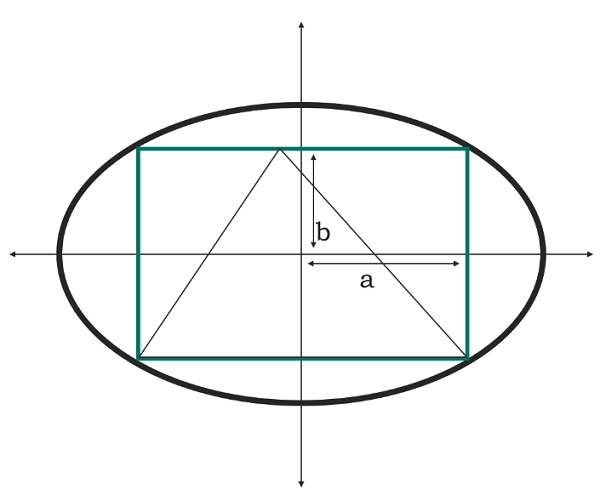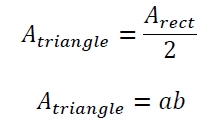# Area of a triangle inscribed in a rectangle which is inscribed in an ellipse In C Program?

Here we will see the area of a triangle which in inscribed in one rectangle and that circle is inscribed in an ellipse. The half of the major and minor axis are ‘a’ and ‘b’ respectively. Suppose the length of the rectangle is ‘l’ and breadth is ‘h’We know that the area of the rectangle in an ellipse is −The area of the triangle is −## Example

#include <iostream>
#include <cmath>
using namespace std;
float area(float a, float b) {
if (a < 0 || b < 0) //if the values are negative it is invalid
return -1;
float area = a*b;
return area;
}
int main() {
float a = 5, b= 2;
cout << "Area is: " << area(a, b);
}

## Output

Area is: 10